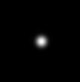The Point Spread Function (PSF) plays an important role in the image formation theory of the fluorescence microscope. It is the main brick that builds up the whole acquired image.

The PSF of an optical device is the image of a single point object (rescaled to make its integral all over the space equal 1). The degree of spreading (blurring) in the image of this point object is a measure for the quality of an optical system.

The PSF is the 'brick' of the image because in incoherent imaging systems such as fluorescent microscopes the image formation process is linear and described by linear system theory. This means that when two objects A and B are imaged simultaneously, the result is equal to the sum of the independently imaged objects. In other words: the imaging of A is unaffected by the imaging of B and vice versa. (The sum is of the light waves which may result in destructive and constructive interference at non-image planes.)Image formation in a confocal microscope

As a result of the linearity property, the image of any object can be computed by chopping up the object in parts, image each of these, and subsequently sum the results. When one chops up the object in extremely small parts, i.e. point objects of varying height, the image is computed as a sum of PSFs, each shifted to the location and scaled according to the intensity of the corresponding point. This is mathematically represented by a convolution equation.

In conclusion: the imaging in the fluorescent microscope is completely described by its PSF. (See image formation).

### In the Huygens software

As the PSF is the basic "brick" of which the images are "built", one should record details at least on the scale of the PSF to gather all the available information. Failing in that spoils any attempt of doing deconvolution, because deconvolution works on the PSF scale. Therefore the ideal sampling density is determined by the optics through the PSF: see the nyquist rate.

The Huygens software, intended for doing deconvolution, enables you to obtain a PSF in two ways:

The best PSF is always the one you measure experimentally, in a sort of calibration of your microscope. If you do not have an experimental PSF, you can use a theoretical one based on the optics of your microscope. For this, it is very important to have the correct microscopic parameters.

Images affected by spherical aberration due to refractive index mismatches are better restored with Huygens Software through the use of depth-dependent PSF's. That is because mismatch distorts PSF.

### Examples

Comparison of measured (left) and theoretical (right) PSF's in a confocal microscope:

The asymmetry and big size of the experimental PSF suggest the presence of lens alignment errors and/or spherical aberration.XY central slice (2×2 µm²)XZ central slice (2×5 µm²)

A Multi Channel image requires a multi-channel PSF for deconvolution. It is quite normal that the PSF differs depending on the channel, specially when each Emission Wavelength follows a different optical path in the microscope (but this does not include Color Shift correction).

The fourier transform of a PSF is called optical transfer function.An example of a three channel PSF

### Calculator

You can use the Nyquist Calculator to see what PSF corresponds to a given Microscopic Parameters set.

### Upcoming Events

 Wed 30 of Sep, 2020 00:00 CEST SVI Huygens imaging Course Sept. 30 - Oct. 2